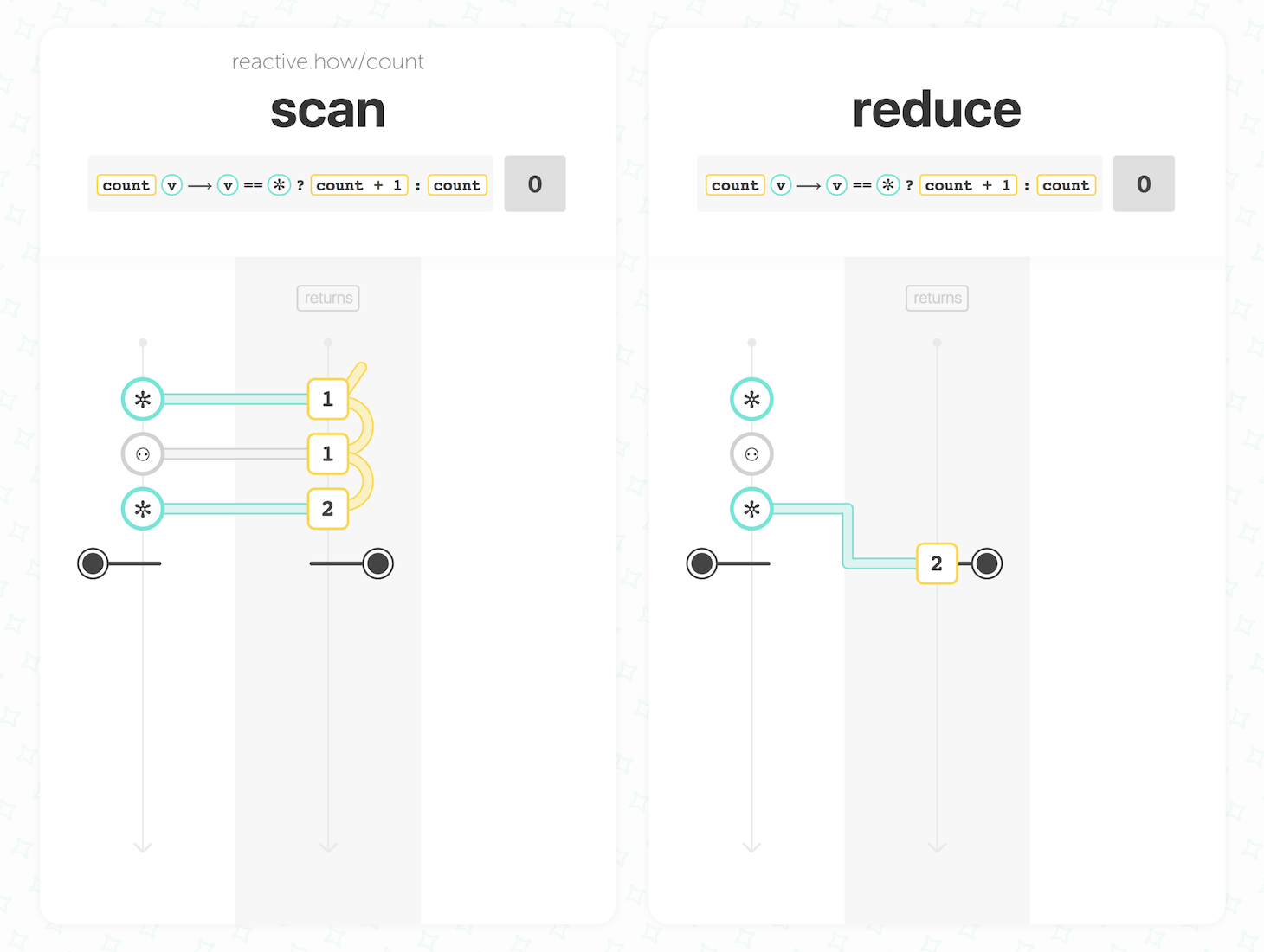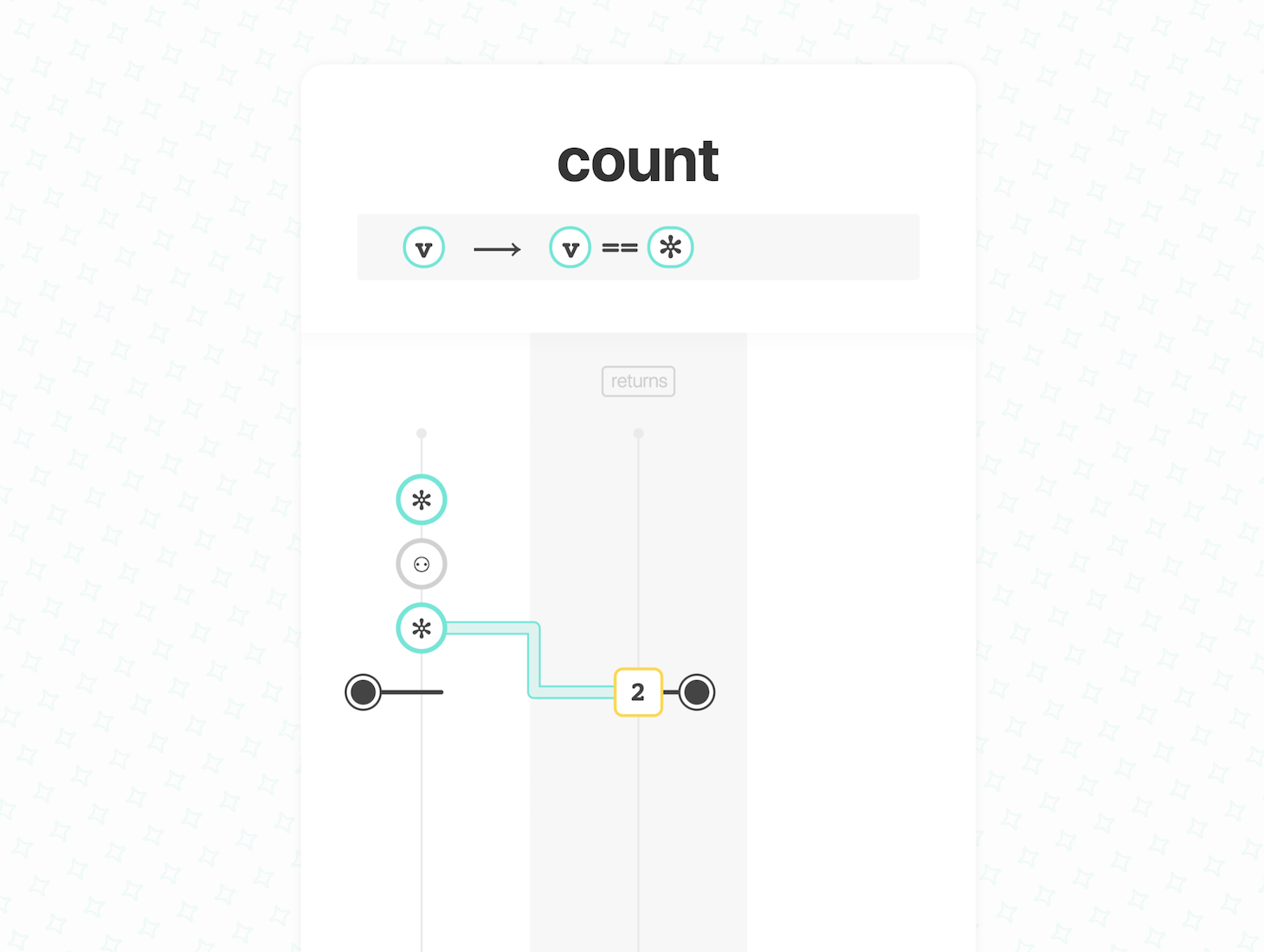reduce

accepts `1` input stream, an `accumulator`, a `seed` (optional)

• The `seed` will be used as an initial accumulation acc
• When the input stream emits a value v:
• v and the latest acc are given to the `accumulator`
• the returned value will be used as the next acc
• When the input stream completes, the output stream emits the last acc and completes

returns a new stream of accumulated values

count

accepts

• (coming soon)

returns

Published on Monday, 19 Feb. 2018
Revised on Monday, 26 Nov. 2018

We learned about `❚ scan` and `❚ reduce`. Here is how you can use them to count the number of values emitted on an input stream:

In scan, the accumulator function accumulated values. In the example above, it only increments the `acc` (accumulation) and the values are ignored. The `acc` is emitted as a count value.

On the one hand, `❚ scan` progressively emits on the output stream the current number of values emitted on the input stream. On the other hand, `❚ reduce` emits the count only when the input stream completes. If the input stream never completes, the output stream will never emit the count.

In RxJS, you actually have a `❚ count` operator. It is equivalent to the `❚ reduce` card seen above:

This is how `❚ count` operates with one input stream:

• When the input stream completes, the output stream:
• emits the number of values emitted on the input stream
• and immediately completes

## Count values that satisfy a predicate

Can you update the cards above to count the number of `✼`?

You can slightly modify the accumulator function to increment the `acc` only when the input value is `✼`:In RxJS, you can simply use `❚ count` with a predicate:This is how `❚ count` operates with one input stream and a predicate:

• When the input stream completes, the output stream:
• emits the number of values emitted on the input stream that satisfied the predicate
• and immediately completes